This function implements decomposition of model predictions with identification of interactions. The complexity of this function is O(2*p) for additive models and O(2*p^2) for interactions. This function works in similar way to step-up and step-down greedy approaximations, the main difference is that in the fisrt step the order of variables is determied. And in the second step the impact is calculated.

break_down(explainer, new_observation, check_interactions = TRUE,
keep_distributions = FALSE)

## Arguments

explainer a model to be explained, preprocessed by function DALEX::explain(). a new observation with columns that corresponds to variables used in the model the orgin/baseline for the breakDown plots, where the rectangles start. It may be a number or a character "Intercept". In the latter case the orgin will be set to model intercept. if TRUE, then the distribution of partial predictions is stored in addition to the average.

## Value

an object of the broken class

## Examples

library("DALEX")
library("breakDown")
library("randomForest")
set.seed(1313)
# example with interaction
# classification for HR data
model <- randomForest(status ~ . , data = HR)
new_observation <- HRTest[1,]
data <- HR[1:1000,]
predict.function <- function(m,x) predict(m,x, type = "prob")[,1]

explainer_rf_fired <- explain(model,
data = HR[1:1000,1:5],
y = HR$status[1:1000] == "fired", predict_function = function(m,x) predict(m,x, type = "prob")[,1], label = "fired") bd_rf <- break_down(explainer_rf_fired, new_observation, keep_distributions = TRUE) bd_rf#> contribution #> (Intercept) 0.386 #> * hours = 42 0.231 #> * salary = 2 -0.216 #> * age:gender = 58:male 0.397 #> * evaluation = 2 -0.019 #> final_prognosis 0.778 #> baseline: 0 plot(bd_rf)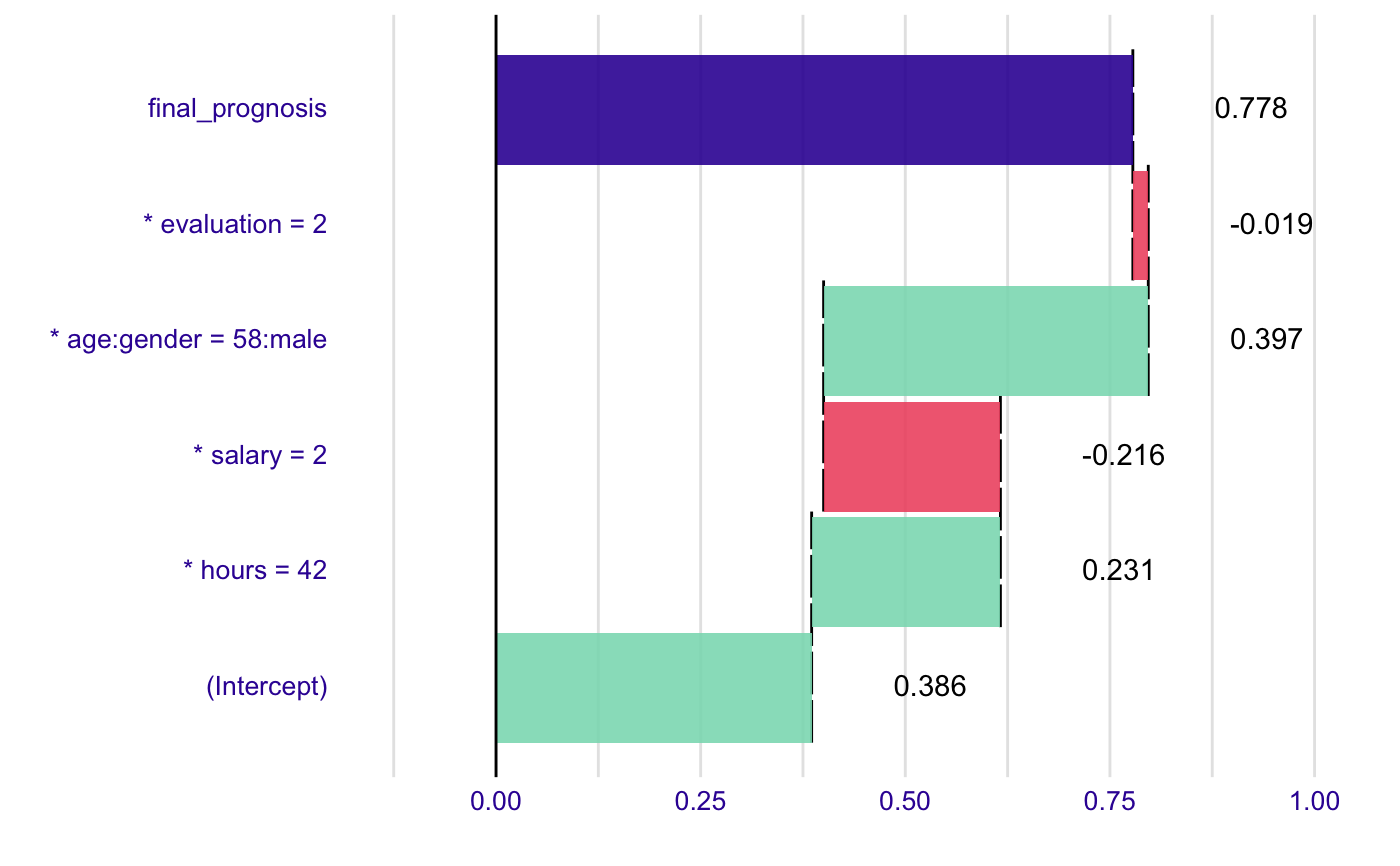plot(bd_rf, plot_distributions = TRUE)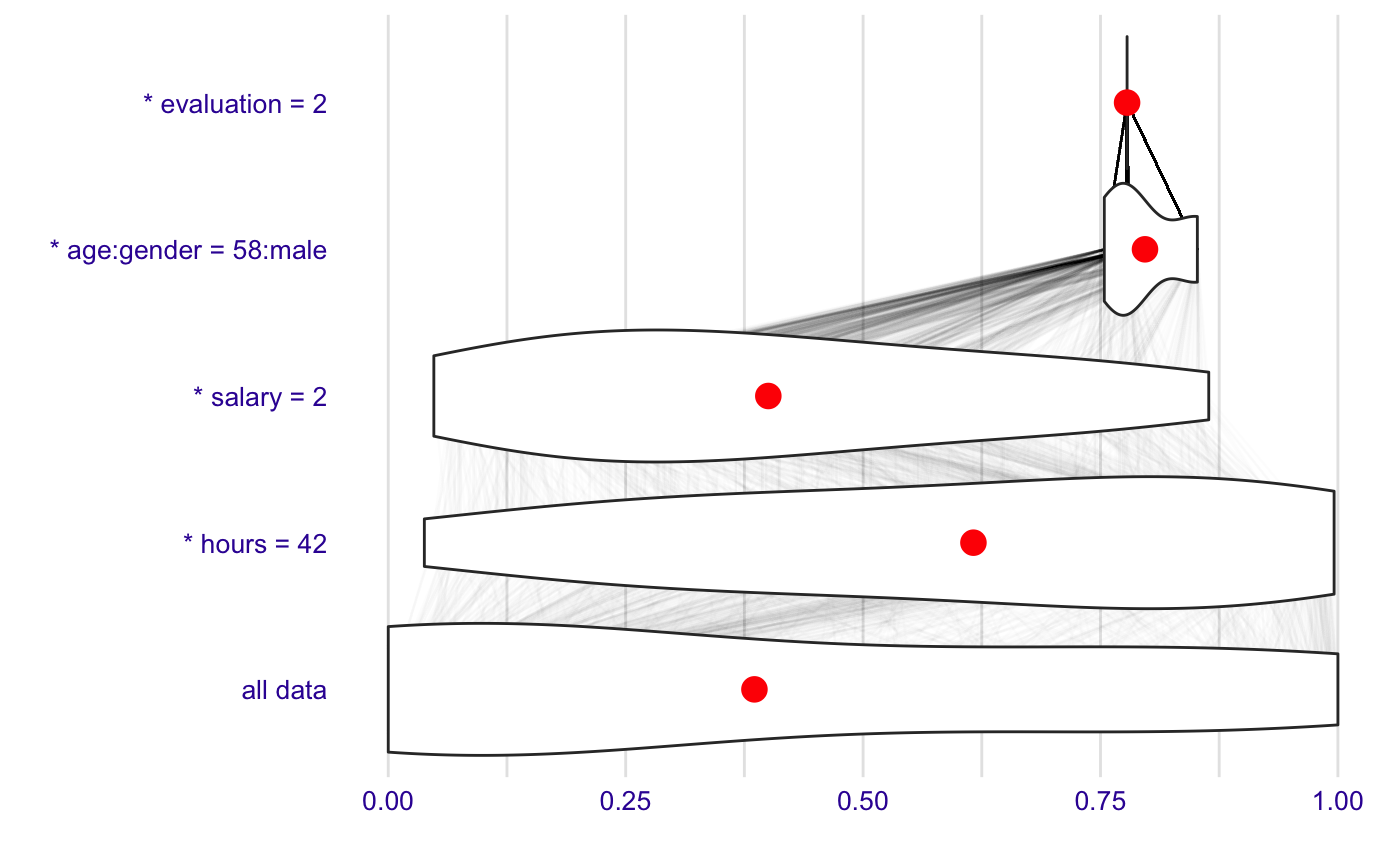bd_rf <- break_down(explainer_rf_fired, new_observation, check_interactions = FALSE, keep_distributions = TRUE) bd_rf#> contribution #> (Intercept) 0.386 #> * hours = 42 0.231 #> * salary = 2 -0.216 #> * evaluation = 2 0.006 #> * age = 58 0.092 #> * gender = male 0.281 #> final_prognosis 0.778 #> baseline: 0 plot(bd_rf)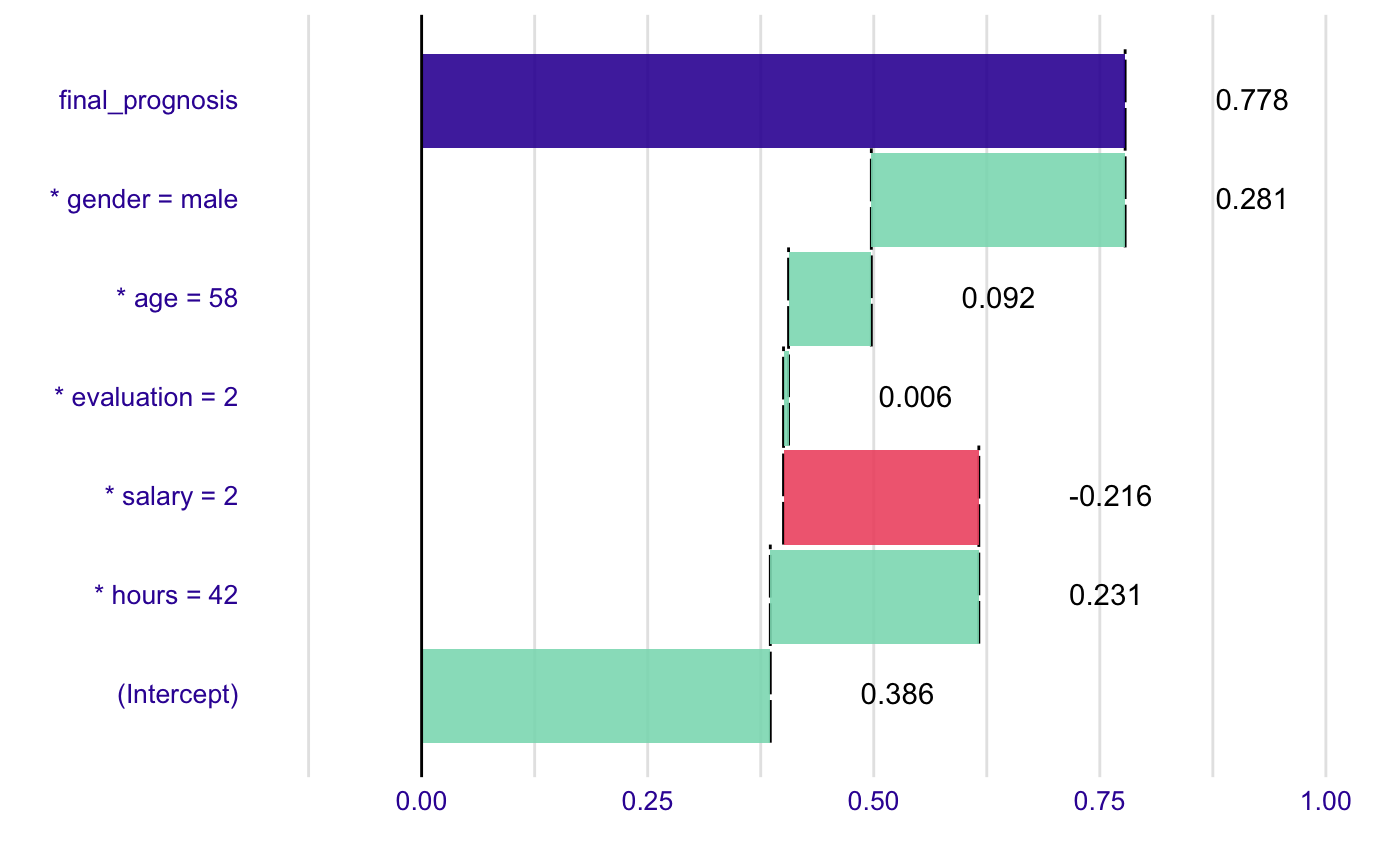# example for regression - apartment prices # here we do not have intreactions model <- randomForest(m2.price ~ . , data = apartments) explainer_rf <- explain(model, data = apartmentsTest[1:1000,2:6], y = apartmentsTest$m2.price[1:1000],
label = "rf")

bd_rf <- break_down(explainer_rf,
apartmentsTest[1,],
check_interactions = FALSE,
keep_distributions = TRUE)

bd_rf#>                            contribution
#> (Intercept)                    3487.722
#> * district = Srodmiescie       1034.737
#> * surface = 130                -315.991
#> * no.rooms = 5                 -163.113
#> * floor = 3                     150.529
#> * construction.year = 2000      -24.021
#> final_prognosis                4169.863
#> baseline:  0 plot(bd_rf)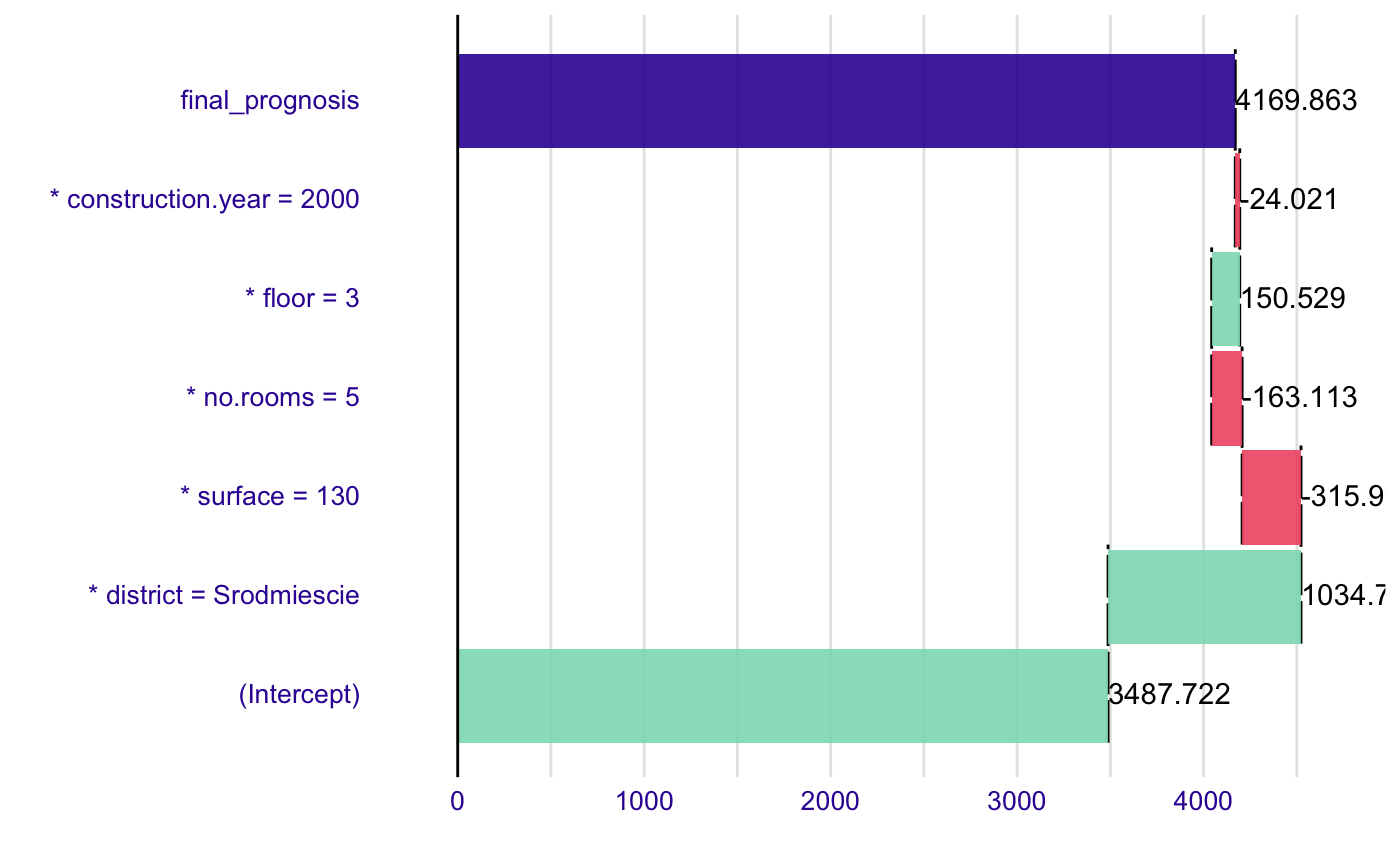plot(bd_rf, plot_distributions = TRUE)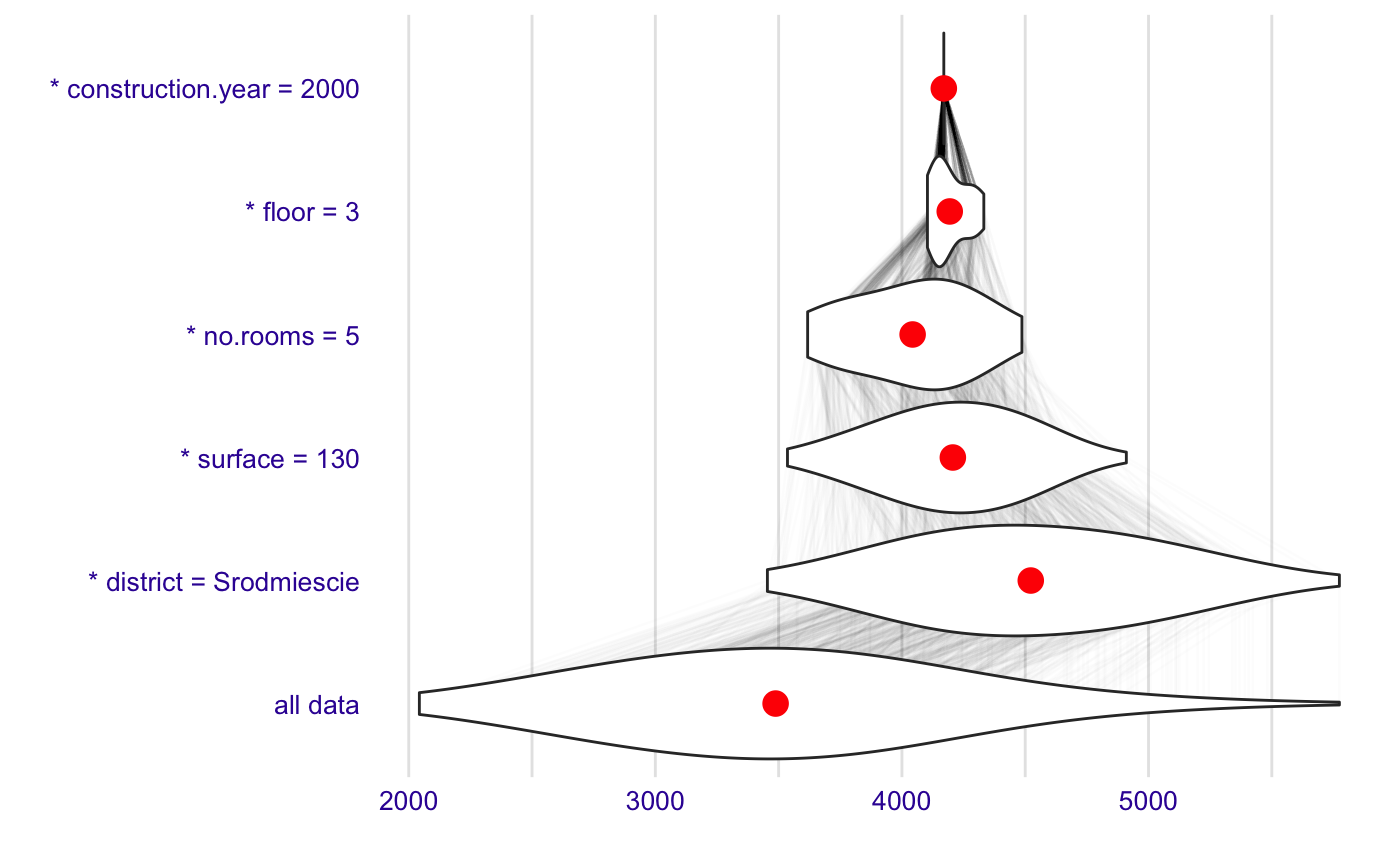`